Documentation

tiledlayout

Create tiled chart layout

Description

example

tiledlayout(m,n) creates a tiled chart layout for displaying multiple plots in the current figure. The layout has a fixed m-by-n tile arrangement that can display up to m*n plots. If there is no figure, MATLAB® creates a figure and places the layout into it. If the current figure contains an existing layout, MATLAB replaces that layout with a new one.

A tiled chart layout contains an invisible grid of tiles that covers the entire figure or parent container. Each tile can contain an axes for displaying a plot. After creating a layout, call the nexttile function to place an axes object into the layout. Then call a plotting function to plot into the axes.

example

tiledlayout('flow') specifies the 'flow' tile arrangement for the layout. Initially, there is only one empty tile that fills the entire layout. As you call nexttile, the layout reflows as needed to accommodate the new axes while maintaining an aspect ratio of roughly 4:3 for all the tiles.

example

tiledlayout(___,Name,Value) specifies additional options for the layout using one or more name-value pair arguments. Specify the options after all other input arguments. For example, tiledlayout(2,2,'TileSpacing','compact') creates a 2-by-2 layout with minimal spacing between the tiles. For a list of properties, see TiledChartLayout Properties.

example

tiledlayout(parent,___) creates the layout in the specified parent container rather than in the current figure. Specify the parent container before all other input arguments.

example

t = tiledlayout(___) returns the TiledChartLayout object. Use t to configure properties of the layout after creating it.

Examples

collapse all

Create a 2-by-2 tiled chart layout, and call the peaks function to get the coordinates of a predefined surface. Create an axes object in the first tile by calling the nexttile function. Then call the surf function to plot into the axes. Repeat the process using different plotting functions for the other three tiles.

tiledlayout(2,2);
[X,Y,Z] = peaks(20);

% Tile 1
nexttile
surf(X,Y,Z)

% Tile 2
nexttile
contour(X,Y,Z)

% Tile 3
nexttile
imagesc(Z)

% Tile 4
nexttile
plot3(X,Y,Z)Create four coordinate vectors: x, y1, y2, and y3. Call the tiledlayout function with the 'flow' argument to create a tiled chart layout that can accommodate any number of axes. Call the nexttile function to create the first axes. Then plot y1 in the first tile. This first plot fills the entire layout.

x = linspace(0,30);
y1 = sin(x/2);
y2 = sin(x/3);
y3 = sin(x/4);

% Plot into first tile three times
tiledlayout('flow')
nexttile
plot(x,y1)Create a second tile and axes, and plot into the axes.

nexttile
plot(x,y2)Repeat the process to create a third plot.

nexttile
plot(x,y3)Repeat the process to create a fourth plot. This time, plot all three lines in the same axes by calling hold on after plotting y1.

nexttile
plot(x,y1)
hold on
plot(x,y2)
plot(x,y3)
hold off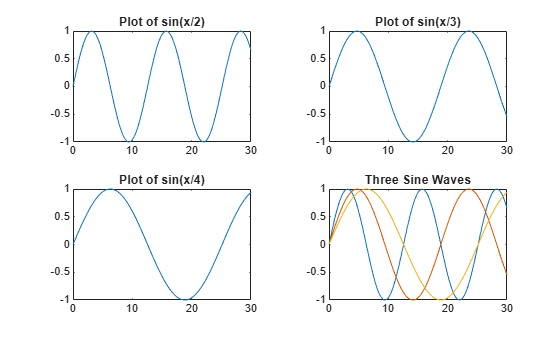Create five coordinate vectors: x, y1, y2, y3, and y4. Then call the tiledlayout function to create a 2-by-2 layout and specify a return argument to store the TileChartLayout object. Call the nexttile function to create an axes object in the next empty tile before calling the plot function.

x = linspace(0,30);
y1 = sin(x);
y2 = sin(x/2);
y3 = sin(x/3);
y4 = sin(x/4);
t = tiledlayout(2,2);

% Tile 1
nexttile
plot(x,y1)

% Tile 2
nexttile
plot(x,y2)

% Tile 3
nexttile
plot(x,y3)

% Tile 4
nexttile
plot(x,y4)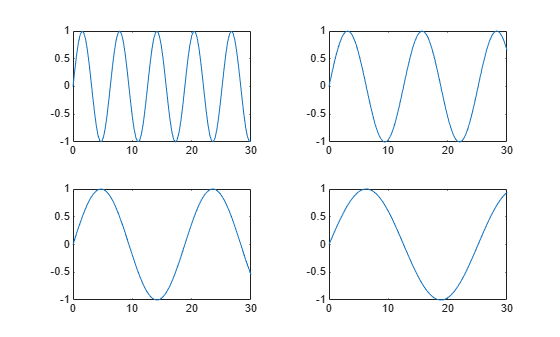Decrease the amount of space between the tiles by setting the TileSpacing property to 'compact'. Then decrease the space between the edges of the layout and the edges of the figure by setting the Padding property to 'compact'.

t.TileSpacing = 'compact';Create a 2-by-2 tiled chart layout t. Specify the TileSpacing name-value pair argument to minimize the space between the tiles. Then create a titled plot in each tile.

t = tiledlayout(2,2,'TileSpacing','Compact');

% Tile 1
nexttile
plot(rand(1,20))
title('Sample 1')

% Tile 2
nexttile
plot(rand(1,20))
title('Sample 2')

% Tile 3
nexttile
plot(rand(1,20))
title('Sample 3')

% Tile 4
nexttile
plot(rand(1,20))
title('Sample 4')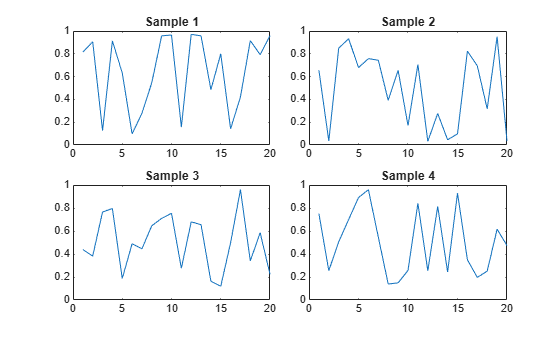Display a shared title and axis labels by passing t to the title, xlabel, and ylabel functions.

title(t,'Size vs. Distance')
xlabel(t,'Distance (mm)')
ylabel(t,'Size (mm)')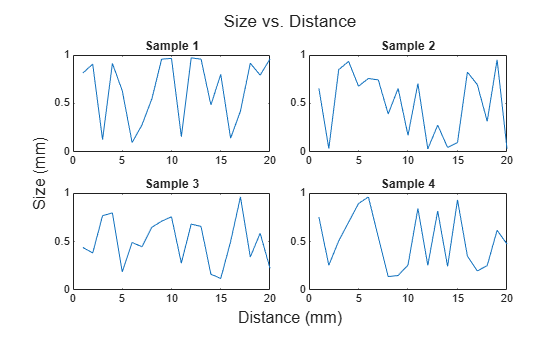Create a panel in a figure. Then create a tiled chart layout in the panel by specifying the panel object as the first argument to the tiledlayout function. Display a plot in each tile.

p = uipanel('Position',[.1 .2 .8 .6]);
t = tiledlayout(p,2,1);

% Tile 1
nexttile(t)
stem(1:13)

% Tile 2
nexttile(t)
bar([10 22 31 43 52])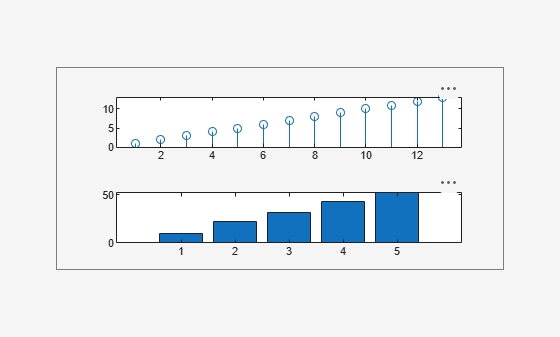Input Arguments

collapse all

Number of rows, specified as a positive whole number.

Example: tiledlayout(2,3) creates a tiled chart layout that has two rows and three columns of tiles.

Number of columns, specified as a positive whole number.

Example: tiledlayout(2,3) creates a tiled chart layout that has two rows and three columns of tiles.

Parent container, specified as a Figure, Panel, or Tab object.

Name-Value Pair Arguments

Specify optional comma-separated pairs of Name,Value arguments. Name is the argument name and Value is the corresponding value. Name must appear inside quotes. You can specify several name and value pair arguments in any order as Name1,Value1,...,NameN,ValueN.

Example: tiledlayout(2,2,'TileSpacing','compact') creates a 2-by-2 layout that has minimal spacing between the tiles.

Note

The properties listed here are only a subset. For a complete list, see TiledChartLayout Properties.

Tile spacing, specified as 'normal', 'compact', or 'none'. Use this property to control the spacing between the tiles. The layout provides space for all decorations, such as axis labels, regardless of the value of this property.

This table shows how each value affects the appearance of a 2-by-2 layout.

ValueAppearance

'normal''compact''none'Padding around the perimeter of the layout, specified as 'normal', 'compact', or 'none'. The layout provides space for all decorations, such as axis labels, regardless of the value of this property.

This table shows how each value affects the appearance of a 2-by-2 layout.

ValueAppearance

'normal''compact''none'